# The Rindler Horizon

## by Greg Egan

This web page was inspired by a discussion on the Usenet group sci.physics.research entitled “The Black Fishing Hole”, in which Edward Green asked for an account of precisely what would happen if someone lowered an object through a black hole’s horizon on a fishing line. I gave a reply describing a slightly different, but related, scenario: that of a constantly accelerating observer in flat spacetime trailing an object behind them. Darryl McCullough made the connection explicit, pointing out that a first-order approximation of the Schwarzschild metric near a black hole’s horizon gives a coordinate system for flat spacetime describing just such a class of observers.

The purpose of this web page, then, is to analyse in detail (using only special relativity) some interesting thought experiments that can be carried out by a constantly accelerating observer, who sees a “Rindler horizon” in spacetime that is very similar to the event horizon of a black hole. This is certainly not a perfect substitute for a full, general-relativistic analysis of the same experiments carried out near a black hole’s horizon. Rather, what it represents is an interesting limiting case: a black hole so massive that the spacetime curvature at its horizon is negligible.

## Preliminaries

Imagine that it’s possible to build a spaceship that constantly accelerates. We don’t really care how it does this; this is a thought experiment in physics, not an exercise in interstellar spacecraft engineering. Because we’re dealing with relativistic velocities, we need to be precise and say that the spaceship undergoes constant proper acceleration: passengers will measure a constant inertial force pushing them towards the back of the ship, whereas observers in motion relative to the ship will necessarily describe its acceleration differently.

If we start out by treating the spaceship as a single point, we can describe its world line in an inertial reference frame with coordinates (t, x) as:

x(τ) = (t(τ), x(τ)) = (sinh(a τ)/a, cosh(a τ)/a)

where τ is proper time measured by a clock in the spaceship (set to zero when t=0), a is the acceleration the passengers feel, and we have chosen coordinates so that x=1/a when t=τ=0. (We are using units where the speed of light is set equal to 1, and this means that whenever we talk about an inverse acceleration being equal to a distance, or vice versa, their product will equal c2 in conventional units. For example 1/g, where g is the gravitional acceleration at the Earth’s surface, corresponds to a distance of 9.18 × 1015m, or about a light-year, while 1/x, where x is one metre, corresponds to an acceleration of 9.18 × 1015g.)

The world line of the spaceship is a hyperbola: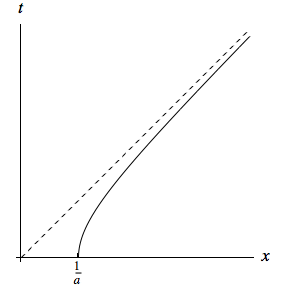This hyperbola can also be described by the equation:

a2x2a2t2 – 1 = 0, or
x·x = 1/a2

where in the second line we’re using the Lorentzian dot product:

(t1, x1) · (t2, x2) = –t1t2 + x1x2

Now, the dashed line in the figure above shows the world line of a flash of light emitted from x=0 at t=0. The spaceship’s world line is asymptotic to that of the flash of light, so the light never catches up with the spaceship. In (t, x) coordinates the two draw ever closer together, but that’s not the case in the spaceship’s own coordinates. If we take a family of inertial reference frames that are instantaneously co-moving with the accelerating spacecraft, each frame will have an orthonormal basis {eτ, es}, where:

eτ = cosh(a τ) et + sinh(a τ) ex
es = sinh(a τ) et + cosh(a τ) ex

For any value of τ, we then have:

(0, 0) = x(τ) – (1/a) es

In other words, the event of the emission of the flash of light is always in the present, and always a distance of 1/a behind the ship. As far as the passengers on the ship are concerned, the flash of light isn’t getting any closer.

Any signal sent from an event that lies to the left of the dashed line in the figure will be unable to reach the spaceship, because that would mean out-racing the pulse of light that itself fails to catch the spaceship. So, as far as the passengers on the ship are concerned, the dashed line constitutes a kind of event horizon, known as the Rindler horizon. Assuming that the spaceship continues to accelerate forever, anything that ends up behind the Rindler horizon will be lost to the passengers forever.

Of course, this is not the same as a black hole’s event horizon in two very important respects. Firstly, it’s always possible to stop the spaceship accelerating, so this horizon’s persistence is a matter of choice, not physical law. Secondly, there is nothing corresponding to a black hole’s singularity to do any actual damage to anything passing through the horizon.

However, the analogy extends this far: a constant force is required to keep the spacecraft a constant distance (by its own measurements) from the Rindler horizon, and, apart from the effects of spacetime curvature (which can be made as small as we like by imagining a black hole with a large enough mass), all the other phenomena (red shift, time dilation and so on) that occur in the vicinity of a black hole’s horizon can be found in the vicinity of a Rindler horizon. There is even an analogue of Hawking radiation, known as Unruh radiation.

We can construct a useful coordinate system that covers the region of spacetime to the right of the horizon. Every straight line of the form:

cosh(q) t – sinh(q) x = 0

will intersect the spaceship’s world line orthogonally to its velocity 4-vector eτ and parallel to the vector es. Now, consider a family of hyperbolas of the form:

x2t2s2 = x · xs2 = 0, or
(t(q), x(q)) = (s sinh(q), s cosh(q))

These will intersect all of the straight lines of constant q orthogonally. So, in the region to the right of the Rindler horizon, we can use q and s as coordinates: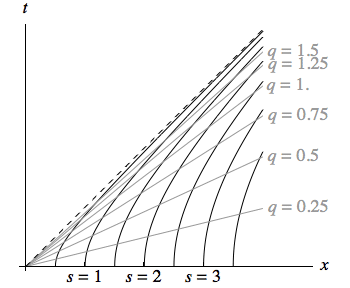Along the x-axis, s agrees with x, and q=0. Although q can be used to parameterise each of the hyperbolas of constant s, it does not correspond to elapsed proper time along these curves; rather, that is given by τ=qs. Along lines of constant q, s measures proper distance.

We can extend our field of orthonormal frames {eτ, es} away from the spaceship itself to arbitrary values of s:

eτ = cosh(q) et + sinh(q) ex
es = sinh(q) et + cosh(q) ex

We can imagine a flotilla of spaceships, each remaining at a fixed value of s by accelerating at 1/s. In principle, these ships could be physically connected together by ladders, allowing passengers to move between them. Although each ship would have a different proper acceleration, the spacing between them would remain constant as far as each of them was concerned. The easiest way to see this is to note that increasing q is equivalent to performing a boost on everything, leaving intervals in spacetime invariant. Another way to describe this is to say that the vector field ∂q generates an isometry of spacetime.

When an object is accelerated in such a way as to preserve the proper distances between its different parts, this is referred to as Born rigid motion (after the physicist Max Born).

## Static situations

The easiest results to calculate involve imagining various observers and objects at fixed positions in terms of our s coordinate, so these are the first things we’ll consider; more interesting possibilities will follow. We will allow light signals to cross between different values of s, but nothing else will move.

### Doppler shift

Suppose a flash of light is emitted from s1 at q=q1, in the forwards direction. Then if an adjacent observer considers the energy of the pulse to be E1, its energy-momentum vector will be:

p = E1 (eτ + es)
= E1 (cosh(q1) + sinh(q1)) (et + ex)
= E1 exp(q1) (et + ex)

By setting q1 to 0 and solving for the value of q2 where the world line for the flash of light hits the hyperbola s=s2, we find:

exp(q2) = s2/s1

and since we can write an expression for p at this second point that takes the same form as the one at (q1,s1), it follows that:

E1s1 = E2s2

where E2 is the energy of the flash measured at s2. This will hold regardless of the particular value of q1. So as we’d expect, light that travels forward away from the Rindler horizon is red-shifted. Similarly, light that travels towards the horizon is blue-shifted.

Another way to obtain this result is to note that the vector field ∂q = seτ is a Killing vector field; that is, as we’ve already noted, it generates an isometry of spacetime. The result then follows from the fact that any geodesic has a constant dot product with a Killing vector field, and so:

E1s1 = s1p · eτ = s2p · eτ = E2s2

Because observers with fixed s coordinates are constantly accelerating, light from distant stars will suffer constantly increasing Doppler shifts, so (unlike the case with black holes) it’s meaningless to talk about a blue shift for light arriving from infinity.Again, let a flash of light (or a radar pulse) pass between two observers at s1 and s2, but this time suppose it is reflected back to the sender, who measures the total elapsed time between emitting the pulse and receiving its reflection.

We can make this calculation simpler by assuming we emit the pulse at the event (–t1, x1) and receive it at (t1, x1); that is, the two events lies on the hyperbola x2t2 = s12 at the same x coordinate and opposite values for the t coordinate, and so by symmetry the event where the pulse is reflected by the ship at s2 will lie on the x-axis at (t, x) = (0, s2).

We then solve for the two requirements:

x12t12 = s12
(x1s2)2t12 = 0

which state that the emission of the pulse (and the reception of its reflection) both occur at s=s1, and that the event of the reflection is connected by a light ray to the emission and reception, and hence has zero space-time separation from those events.

These equations are solved by:

x1 = (s12 + s22) / (2 s2)
t1 = ±(s12s22) / (2 s2)

What is the elapsed time measured on the ship at s1 between sending the pulse and receiving the reflection from the ship at s2? The q coordinate of the event (t1, x1) is given by:

cosh(q1) t1 – sinh(q1) x1 = 0
tanh(q1) = t1 / x1 = ±(s12s22) / (s12 + s22)
q1 = ±log(s1/s2)

The proper time measured on any ship in the flotilla is given by the product of its fixed s coordinate and the change in the q coordinate, so the elapsed time is given by:

τ = 2 s1 |log(s1/s2)|

For non-accelerating observers, we would expect half the elapsed time to measure the proper distance between the emitter and the reflector at the time of the reflection. But τ/2 will be greater than |s1s2|, and as s2 approaches zero, it will tend to infinity.

So, in the flotilla, even though the ships remain at a fixed proper distance from each other, as measured in instantaneously comoving frames, that distance can not be found with the kind of radar range-finding methods that are applicable in non-relativistic situations, or when the bodies in question are moving at constant velocities in flat space-time.

### Suspended string

Suppose we fix the s coordinate of one end of a string and let it dangle above the Rindler horizon. We’ll call the s coordinate of the fixed end s2, and that of the free end s1. Because the tension in the string becomes zero at the free end, it’s easier to deal with the boundary conditions if we describe everything in terms of s1.

We will assume that the string obeys Hooke’s law exactly (that is, each part of it stretches in proportion to the force applied) even as the tension it experiences grows arbitrarily large; this is unrealistic, but it is the simplest assumption we can make that’s compatible with special relativity, which does not permit perfectly inelastic bodies. (There are some further restrictions needed, which we’ll describe shortly.) We’ll call the constant of proportionality k, and we will assume that the string has a constant proper mass density of ρ per unit of unstretched length. We’ll use a parameter λ to describe the unstretched distance along the string, starting from the free end.

Rather than working explicitly with the tension in the string, we will compute everything in terms of pressure, since this is a more common starting point for the stress-energy tensor that we’ll need to write down; we simply need to remember that the pressure will be negative. We start by expressing the quantities we need in terms of n(s), the factor by which the string has been compressed (which will be less than 1 when the string has been stretched):

n(s) = dλ(s)/ds
p(s) = k (1 – 1/n(s))
u(s) = (k/2) (1 – 1/n(s))2

Here p(s) is the pressure, and u(s) is the density of potential energy stored in the string, per unit of unstretched length. We can rewrite n(s) and u(s) in terms of the pressure:

n(s) = k / (kp(s))
u(s) = p(s)2 / (2k)

Now, if we work in just one dimension of space and one of time, the stress-energy tensor for the string will be:

T = n(s) (ρ + u(s)) eτeτ + p(s) eses

where the first term comes from the total energy density of the string, and the second from the pressure. In order for this stress-energy tensor to satisfy the weak energy condition — the requirement that the energy density measured by any observer is non-negative — the tension in the string can not be greater than the energy density.

If we compute the divergence of T, which must be zero to conserve energy-momentum, the τ component is identically zero, while the s component gives us the equation:

(1/s) n(s) (ρ + u(s)) + (1/s) p(s) + p'(s) = 0

Since the proper acceleration of any object with a fixed s coordinate is 1/s, this equation is almost just F = m a for an element of the string occupying one unit of s, with p'(s), the rate of change of pressure in the string, being the opposite of the force on the element, and n(s) (ρ + u(s)) giving its total inertial mass. However, the extra term (1/s) p(s) is necessary to conserve momentum; this arises because the flow of momentum associated with the pressure is changing its direction in spacetime as the element of the string accelerates, turning it into a density of momentum. (Earlier versions of this page, prior to 11 December 2006, neglected this term.)

If we express everything in terms of p(s), we obtain the differential equation:

s (p(s) – k) p'(s) + (1/2) p(s)2k p(s) – k ρ = 0

This is solved by:

p(s) = k (1 – (1/K) √(1 + K2s1/s))

where K2=k/(2ρ), and p(s1) = 0 as required by our boundary conditions. Because √(k/ρ) is the speed of sound in the string (in the small-amplitude limit, for the relaxed material), we should impose the restriction K2 < 1/2, in order that the speed of sound does not exceed 1, the speed of light.

Here are some plots of –p(s)/k versus s/s1 for K ranging from 0.1 to 0.7.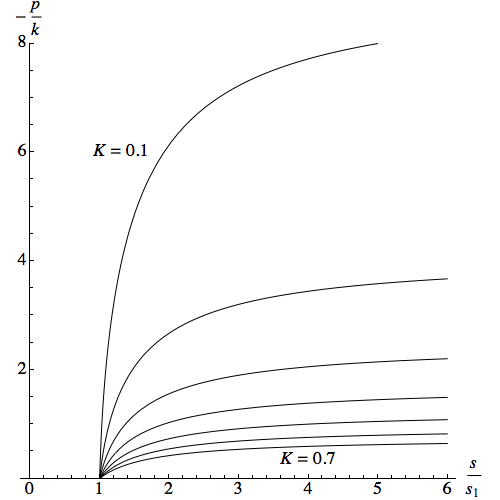Note that for given values of K and k, the tension in the string is bounded, regardless of its length:

p(s) < k (Q/K – 1)

where we’ve defined Q2=1+K2. It turns out that this bound is precisely what’s needed to guarantee that the stress-energy tensor satisfies the weak energy condition! The same condition can be expressed more concisely as:

ds/dλ = 1/n(s) < Q/K

In other words, the string can not be stretched by a factor greater than Q/K. For K=1/√2, Q/K=√3; however, for smaller K this limit grows larger, so there is no universal bound that must apply to all strings.

N.B. We have already restricted the value of K so that the speed of sound in the relaxed material does not exceed the speed of light. However, as shown on this page, the speed of sound will vary with the tension, and for a string under uniform tension, the speed of sound will reach the speed of light when it is stretched by a factor of Q/(√3 K), or just 57% of the stretching that would violate the weak energy condition. We can’t expect the same result to hold exactly for our suspended string (which will have a complicated dispersion relation, with different frequencies of sound travelling at different speeds), but it’s highly likely that the hyperelastic model will become physically impossible at some point significantly below the stretch factor of Q/K. Computing that point exactly is beyond the scope of this analysis, so we will present solutions that obey the hyperelastic model all the way up to Q/K, but these results need to be viewed with the caveat that even idealised materials will necessarily depart from them at sufficiently high tension.

We can find dλ(s)/ds from our solution for the pressure, and then integrate to obtain λ(s):

dλ(s)/ds = K √(s / [Q2ss1])
λ(s)/s1 = (K/Q3) [log[Q √(s/s1) + √(Q2s/s1 – 1)] – log[Q + K] + Q [√(s/s1 (Q2s/s1 – 1)) – K]]

Below are some plots of the solution, for K ranging from 0.1 to 0.7.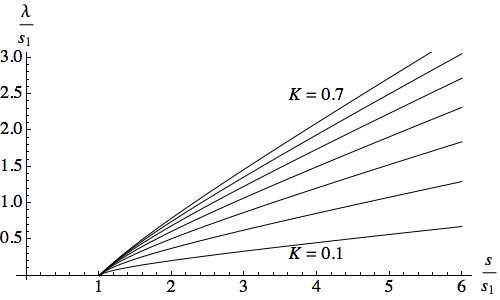Note that as s/s1 → ∞, λ(s) is asymptotic to (K/Q) s, in the sense that the ratio of the two expressions tends to 1 (the difference between them does not tend to zero, but grows smaller as a proportion of the expressions themselves).

We can numerically invert this solution to see how the locations of the top (s2) and bottom (s1) ends of the string are related, for a string of unstretched length L:Each curve starts at s2/L = Q/K, then descends to a minimum value for s2, below which there can be no equilibrium. Depending on how easily stretched the string is, this can occur when the top of the string is much further from the horizon than the unstretched length L, and even for the maximum value of K the minimum for s2/L is approximately 1.72. For values of s2 greater than this minimum but less than Q/K, there are two equilibrium positions for s1: a higher one, which will be stable, and a lower one, which will not.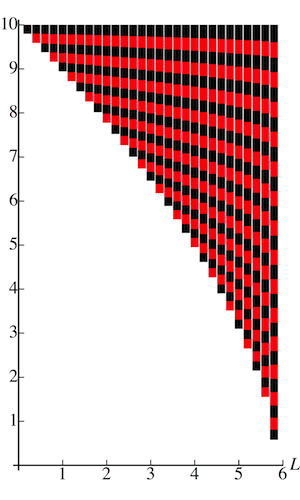It follows that, for a given value of K (which sets the stiffness of the string) and a given value of s2 (the height of the top of the string), there is a maximum unstretched length L for a string suspended in stable equilibrium. For strings with the maximum possible stiffness (i.e. with a speed of sound equal to the speed of light), this is approximately 0.58 s2.

The picture on the left shows strings of the maximum possible stiffness of various unstretched lengths L, all suspended from the same height above the Rindler horizon, in stable equilibrium. Segments of equal unstretched length are marked in bands of alternating colour.

Although we haven’t studied any dynamic processes here, it seems safe to conclude that:

• if you suspend a given string from too low a point, it will be subject to unbounded stretching (and any real string would subsequently break);
• if you anchor the string from a safe point but with s2/L < Q/K, and then apply an extra force to drag the bottom end down past the point of unstable equilibrium, the string will be unable to snap back up, and will suffer the same unbounded stretching.

If these results seem hard to believe, don’t forget that we’re talking about extremely powerful accelerations. The acceleration associated with s2 = 10 metres is 9.18 × 1014g, and although it’s obvious that you can’t trail anything longer than 10 metres behind you at that acceleration, we’ve found that even a string just 6 metres long would be doomed.

## Free fall

Suppose a passenger named Adam on our flotilla of spaceships, travelling at an s coordinate of s0, steps off the ship. Without loss of generality we can choose coordinates so that he does this at (t, x) = (0, s0). His free-falling world line is then just x=s0, and his proper time will correspond to the t coordinate of our global inertial frame. At t=s0, he will pass behind the Rindler horizon, after which no signal from him can ever catch up with any part of the flotilla.

It’s worth noting Adam’s rates of change for the coordinate s = √(x2t2), which are just the rates of change with respect to t:

ds/dt = –t/s
d2s/dt2 = –(s2 + t2) / s3

Now suppose that Adam’s friend Eve was travelling with him at s=s0, and remains on the same ship. There is no finite q coordinate for any point on the Rindler horizon, which means there is no time for Eve when, in her co-moving inertial reference frame, Adam passes through the horizon. In that sense, Eve could claim that Adam never reaches the horizon as far as she’s concerned. However, not only is it clear that Adam really does cross the horizon, there is a time τcrit for Eve after which any signal she sends to Adam will reach him only after he’s on the other side.

It’s easy to compute this time. The event of Adam reaching the horizon is (t, x) = (s0, s0), and the null line passing through this event for light coming back towards Adam from the direction of the ships consists of points of the form (t, x) = s0(1–z, 1+z). Eve’s world line is s0(sinh(q), cosh(q)), and so it intersects that null line when:

sinh(q) + cosh(q) = 1–z + 1+z
exp(q) = 2
q = log(2)
τcrit = s0 log(2)

After a time of τcrit has passed for Eve, she must concede that it’s too late for her to send Adam a message asking him to hitch a ride and catch up with the ship, since every signal she now sends will be received by him on the other side of the horizon.Suppose Adam decides to tie a rope around his waist when he steps off the ship, but Eve agrees to feed out the rope in such a way that Adam remains in free fall. Is this possible? Clearly it is, because we can imagine a rope of arbitrary length sitting motionless in our (t, x) coordinates, and all Eve has to do to keep her and Adam’s rope slack is to feed it out in such a way that it matches that reference rope. This will require Eve to give the section of rope she is dispensing a velocity equal and opposite to her own ordinary velocity in the (t, x) frame, which is tanh(τ/s0). If Eve sticks to her notion of simultaneity then she’ll never admit that Adam has passed through the horizon, so her task is endless (and the velocity she needs to give the rope will asymptotically approach the speed of light), but if she takes a more sensible approach and concedes that after a time of τcrit has elapsed there’s no hope of hauling him back on to the ship, she will have fed out a length of just s0 [cosh(τcrit/s0) – 1] = s0/4 before reaching that point. The velocity at which she will be dispensing the rope at τcrit will be tanh(τcrit/s0) = 3/5.

We should note that the velocity Eve must give the rope at the point where she is unreeling it does not equal the rate at which the total proper length of rope she has dispensed is increasing. The latter is equal to v/√(1–v2), or in this case, sinh(τ/s0). At τcrit, this rate is sinh(τcrit/s0) = 3/4.

If Eve clamps down on the rope after τcrit (or in fact somewhat earlier, depending on the speed of sound in the rope), then the wave of tension passing down the rope will not reach Adam until he is behind the horizon, and however powerful an acceleration this gives him, he will not be able to pass back through the horizon, because that would mean travelling faster than light.

When will the rope break? There is no particular time when this must happen; it will depend on the rope’s actual properties (though as we’ve noted earlier, the weak energy condition does put an upper limit on the amount by which any given rope can be stretched). The tension in the rope does not become infinite just because it crosses the horizon. What a clamped rope spanning the horizon does imply, though, is that it must eventually break, because the portion of it in the neighbourhood of the horizon will always be moving backwards relative to the ship.

### Dust trail

Suppose that Eve opens a box of dust and lets it waft freely out of the ship at a constant rate; in other words, by her clock the time between one particle and the next leaving the ship is constant. The world lines of these dust particles will be tangents to the ship’s world line. They are the straight grey lines, labelled by values of d, in the spacetime diagram below.The curves on the diagram that cross between the dust’s world lines, labelled by values of w, have been chosen to be orthogonal everywhere to those world lines. Someone like Adam, who jumped ship, would consider these curves (locally) to be surfaces of simultaneity. It’s interesting to ask what he would consider the density of the dust trail to be.

To do this, we need to describe these tangent lines and the curves orthogonal to them in more detail. We’ve chosen to label the world lines of the dust particles by the ship time at which they were emitted, so where these lines commence at the ship’s world line, d=τ. We have also labelled the curves of constant w by the ship time when they meet the ship’s world line.

If we wish to find the d coordinate of some event x, suppose that x lies on the world line of the dust particle that left the ship at the event p = s0(sinh(d(x)/s0), cosh(d(x)/s0)). Then we know that xp is the tangent to the ship’s world line at p, and since the ship’s world line is orthogonal to any line that connects it to the origin (the lines of constant q in our (q, s) coordinates), xp must be orthogonal to p itself:

p · (xp) = 0
p · x = p · p = s02

Define k(x) to be the proper time that has elapsed for the dust since it left the ship, i.e.

k(x)2 = –(xp) · (xp) = –(x · x – 2p · x + p · p) = s02x · x
k(x) = √(s02x · x)

If we define:

d(x) = s0 [ arctanh(t/x) – arctanh(k(x)/s0) ]
= s0log[(x+t) / (s0 + k(x))]
= s0log[(s0k(x)) / (xt)]

then it’s not hard to show that p = s0(sinh(d(x)/s0), cosh(d(x)/s0)) satisfies p · x = s02.

Note that on the ship’s world line, x · x = s02 and k(x) = 0, so:

d(x) = s0log[(x+t) / s0] = s0log[exp(q)] = s0q = τ

If we define:

w(x) = k(x) + d(x)

then on the ship’s world line we will have w(x) = d(x) = τ. For generic events x, since k(x) is the proper time for the dust particle since it left the ship, and d(x) specifies the value of τ when it left, w(x) is the total proper time for the dust since it passed through the event (t, x) = (0, s0), including both its shipboard time and its time in free fall.

Now, if we compute the derivatives of d(x) and w(x) with respect to t and x, we get:

t d(x) = s0(xs0t / k(x)) / x·x
x d(x) = s0(s0x / k(x) – t) / x·x
t w(x) = (s0xk(x) t) / x·x
x w(x) = (k(x) xs0t) / x·x

It’s easy to see that the gradients of these functions are orthogonal.

The magnitude of the gradient of d(x) turns out to be s0/k(x). Since k(x) is the proper time that has elapsed since the dust left the ship this is singular at the instant the dust leaves the ship, which makes sense because we’re modelling the source of the dust trail as a single point. Since the magnitude of the gradient corresponds to the particle density of the dust trail, our result shows that the spacing between the particles grows linearly with time.

We’ve already seen that the proper time for any free-falling object to reach the Rindler horizon is s0, so as the dust trail crosses the horizon, k(x) = s0 and the local particle density becomes equal to 1. In other words, if particles were leaving the ship at a rate of 1 every second, they would now be spaced at one every light-second! If we imagine that this is some kind of smart dust made of particles that can signal to each other, then if a particle reaches the horizon and sends a light signal back to the one coming after it, the receiving particle will get the message at precisely the moment when it, too, arrives at the horizon.

The dust-trail scenario can easily be generalised to the case where the dust is thrown out backwards with a speed of –v, by replacing s0 in the preceding analysis with s0 / √(1 – v2). Then by the time the dust has reached s=s0, it will have a velocity relative to the flotilla of –v.

## Dynamic situations

We’ve seen that it requires an acceleration of 1/s to maintain a fixed distance of s from the horizon, an acceleration that obviously grows without bounds as you approach the horizon itself. We’ve also seen that there is no problem with free-falling right through the horizon (apart from the fact that you can’t cross back).

This leads to the question of exactly what kinds of motion, apart from free fall, can take you through the horizon. To what degree can you control your fall, without requiring infinite acceleration?

### Constant velocity with respect to the flotilla

Suppose Adam decides that, rather than free-falling through the horizon, he wants to use a rocket backpack to control his descent. His goal is to maintain a constant ordinary velocity of –v relative to each ship in the flotilla that he passes, all the way down to the horizon itself. Of course any real flotilla must end at some finite distance above the horizon, but Adam plans to follow the same criterion whether there’s an actual ship beside him or not.

At any event (t, x) with x > t, the q coordinate is arctanh(t/x), so the 4-velocity of the flotilla ship passing through this event is:

uflotilla = (cosh(q), sinh(q)) = 1/√(x2t2) (x, t)

What we want is a world line for Adam that has a constant dot product with this vector field of uAdam · uflotilla = –1/√(1–v2). It turns out that we can describe such a world line in (t, x) coordinates by the equation:

x + t = A2 (xt)–(1–v)/(1+v)

where A2 is a constant that sets the position of the world line without changing its properties of interest. We can write the (t, x) coordinates of this world line in terms of a parameter w = xt, while being careful to keep in mind that this parameter is not proper time along the world line:

(t, x) = (1/2) (A2 w–(1–v)/(1+v)w, A2 w–(1–v)/(1+v) + w)

The tangent vector with respect to w is then:

w(t, x) = –(1/2) ((1–v)/(1+v) b(w)2 + 1, (1–v)/(1+v) b(w)2 – 1)
where b(w) = A w–1/(1+v)

We can normalise this vector to a squared length of –1 to get Adam’s 4-velocity, also taking account of the fact that ∂w(t, x) points backwards in time so we need to reverse it:

uAdam = 1/[2√(1–v2)] ((1–v) b(w) + (1+v) / b(w), (1–v) b(w) – (1+v) / b(w))

If we note that, along Adam’s world line:

x + t = w b(w)2, and
x2t2 = w2 b(w)2

then it’s not hard to show that:

This verifies our claim that the ordinary velocity Adam and the flotilla ship will measure for each other has a magnitude of v.

Finding Adam’s proper acceleration is then just a matter of calculating the rate of change of his 4-velocity along his world line with respect to his proper time, and taking the magnitude of the resulting vector. This turns out to be:

In other words, his acceleration is just as singular in s as that required to remain stationary with respect to the horizon. In fact the acceleration for any given value of s is actually greater when v is non-zero!

So Adam’s goal of passing right through the horizon in this manner is physically impossible; even though he’s not trying to remain stationary, his rocket would still have to provide an infinite acceleration as he crossed the horizon.

What does this tell us about lowering an object on a string? We can’t say that we’ve ruled out the possibility of feeding out string from a reel at a constant rate, because the unavoidable elasticity of the string means the object being lowered need not end up moving at a constant velocity relative to the flotilla. There is also the further complication of the difference between clocks at the top and bottom of the string. So we can only say that any scheme that aims to adjust the rate at which the string is fed out in such a way as to cause the object itself to be lowered at a constant rate compared to the flotilla (according to its own clock) will necessarily fail as the object approaches the horizon.

### Finite proper acceleration at the horizon

Now that we can see that Adam must accelerate with respect to the flotilla as he passes through the horizon, let’s try to estimate the kind of acceleration that’s necessary.

The world line:

(t, x) = (1/a)(1 + sinh(a τ), cosh(a τ))

is a world line of constant proper acceleration a, and it crosses the horizon s = √(x2t2) = 0 at τ=0. To lowest order in τ, we can approximate it as:

(t, x) = (1/a)(1 + a τ, 1 + a2 τ2 / 2)

and then to lowest order in τ:

s = √(–2τ/a)
τ = –a s2/2

The sign inside the square root here reminds us that we’ll have to consider everything for small negative values of τ, not positive ones, because the s coordinate is not defined on the far side of the horizon.

The derivatives of s with respect to τ are then:

ds/dτ = –1/(a s)
d2s/dτ2 = –1/(a2s3).

So in order to achieve a finite proper acceleration at the horizon, Adam must accept that he will see the s coordinate of the flotilla changing with respect to his proper time in this fashion. Note the inverse square relationship: the lower Adam’s proper acceleration, a, the greater the coefficient in the leading term for the second derivative of s.

### Descending strings that are static in another frame

We can get our hands on one class of exact solutions for elastic strings descending through the horizon by the simple expedient of taking a string that is static in one flotilla’s (q, s) coordinates, and then viewing it from the perspective of a different flotilla. We can obtain the world lines for the ships in the new flotilla simply by shifting the origin of the (t, x) coordinates. So if the world lines in our “reference” flotilla are:

(t, x) = (s sinh(q), s cosh(q))

then those in the second flotilla, which we’ll call the “primed” flotilla, will be:

(t, x) = (A + s' sinh(q'), B + s' cosh(q'))

for some constants A and B. Of course, if the event (A, B) lies above (or to the right of, in a conventional spacetime diagram) the Rindler horizon of the reference flotilla, the entire primed flotilla will also remain above the horizon, so those values of A and B will be of no use to us.

We will suppose that there is a stationary string suspended above the horizon of the primed flotilla, with the bottom of the string at a coordinate of s'1. If we wish to make use of this solution in the reference flotilla, we can pick a location s2 and imagine that we are feeding out the string from that point. Suppose the bottom of the string passes s2 at τ=0, and its ordinary velocity is measured by Eve, riding in the reference flotilla at s2, to be –v0, heading towards the horizon. We can then solve for A and B:

A = s'1 γ0 v0
B = s2 – γ0 s'1
where γ0 = 1 / √(1–v02)

Of course the velocity of the string adjacent to Eve (or being unreeled by her, if she is matching the solution by her own actions) will not remain constant. The expression for the velocity is:

v(τ) = – √[g(τ)2s'12s22 + 2 s'1 s2 γ0] / [g(τ) + s2]
where g(τ) = s'1 cosh(τ/s2 – log(Γ0)) – s2 cosh(τ/s2)
and Γ0 = γ0 (1–v0) = √[(1–v0) / (1+v0)]

The bottom of the string passes through the reference flotilla’s horizon at:

t = x = [Γ0s2(2 s'1γ0s2)] / [2 (s'1 – Γ0s2)]

and it follows that after

τcrit = s2 log([Γ0(2 s'1γ0s2)] / [s'1 – Γ0s2])

no influence from Eve can reach the bottom of the string before it goes behind her Rindler horizon. At τ = τcrit (as at all finite values for τ) everything Eve measures about the string — the tension, the rate at which she is feeding it out, and the total length she has unreeled — will all be finite. However, if Eve tries reeling the string back up after this time, the bottom will remain on the far side of the horizon, and the string will eventually break.The function v(τ) which describes the velocity at which Eve must unreel the string is plotted below for the case s'1 = s2 = 1, for various values of v0.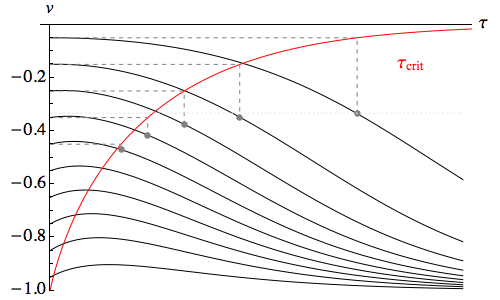Also plotted is τcrit as a function of v0 (the red curve). Naturally the slower the string is being unwound, the longer it is until the bottom of the string has effectively been lost behind the horizon.

The velocity at which the string is being unwound by Eve when this happens, vcrit), is shown on the graph for a few different values of v0. For s'1 = s2, in the limit of v0 → 0, vcrit) → –1/3. In other words, for a string that starts out being unreeled slowly, there is a ceiling of roughly one third lightspeed on the speed at the point of unreeling at τcrit.

## String unreeled at a constant rate

Although the descending string described in the previous section has the advantage of an exact, analytical solution, it’s a rather artificial set-up. What we’d really like to understand is what happens when a string is unreeled at a constant rate.

What we’ll take this to mean is that a constant unstretched length of string, R, is unreeled per unit of proper time from some fixed point on the flotilla. To understand what this entails, it’s worth looking first at the Newtonian solution for a string being unreeled at a constant rate by an observer undergoing constant acceleration. The x coordinate of an element of string then obeys the linear wave equation:

t,tx(λ,t) = w2λ,λx(λ,t)

where w is the speed of sound in the string; in terms of our previous parameters:

w2 = 2 K2 = k / ρ

The appropriate solution is a quadratic in λ and t:

x(λ,t) = x0 + λ – R t + (a/2) (λ2 + w2 t2) / (w2 + R2)

If we put λ = R t, we get:

x(R t, t) = x0 + (a/2) t2

which is just the x coordinate of the constantly accelerating observer who is unreeling the string.

The derivatives of this solution are easily computed:

λx(λ,t) = 1 + a λ / (w2 + R2)
λ,λx(λ,t) = a / (w2 + R2)
tx(λ,t) = – R + a w2 t / (w2 + R2)
t,tx(λ,t) = a w2 / (w2 + R2)

The second derivatives make it plain that the wave equation is satisfied, while the first derivative with respect to λ shows that at the bottom of the string, where λ = 0, stretched and unstretched length change at the same rate, i.e. there is no tension in the string. The tension depends only on λ and grows linearly along the string. Explicitly, if p(λ) is pressure:

p(λ)/k = a λ / (w2 + R2)

The first derivative of x with respect to t shows us that the ordinary velocity of the string, relative to the constantly accelerating observer dispensing the string (whose own velocity at time t is a t), will be:

v = – R + a w2 t / (w2 + R2) – a t = – Ra R2 t / (w2 + R2)

This is independent of λ, but if we want the relative velocity of the string as it is being dispensed, we set t = λ / R, and then:

vdispensed(λ) = – Ra R λ / (w2 + R2) = –Rλx(λ,t)

It might seem odd to think of the string’s velocity relative to the person unwinding it not being constant, despite the fact that a constant amount of unstretched length is being unreeled. However, unless some mechanism completely isolated the portion of the string wrapped around the reel from the tension in the rest of the string, the string on the reel would be stretched to some degree, so the reel would be rotating ever faster to dispense unstretched length at a constant rate. If a tension-isolating mechanism was used, the string would be accelerated within that mechanism, as it underwent the transition from zero tension to full tension.

The fact that the velocity of the string is independent of λ and the tension is independent of t shows us that, in the Newtonian case, the “artificial” trick of using a static string suspended by one accelerating observer and then viewing it in a different accelerating frame gives us exactly the same result as unreeling unstretched length at a constant rate! The tension in a string that is static in a frame with acceleration a' is given by:

p(λ)/k = a' λ / w2

So we can match the tension exactly if we set:

a' = a w2 / (w2 + R2)

Our “unreeling observer” with acceleration a will overtake the frame with the static string and acceleration a', but if they start out with the bottoms of their strings coinciding — and with the “unreeling observer” already moving with speed R relative to the static string — then the tension and velocity of the two strings will match all along their length.

The plot below shows the trajectories of elements of the string separated by equal increments of unstretched length λ, along with the trajectory of the unreeling point.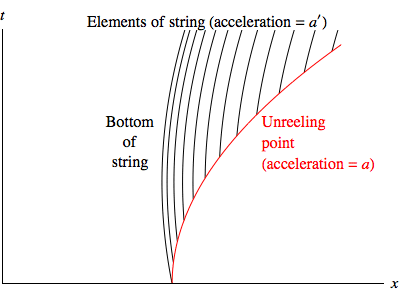Unfortunately, the Newtonian relationship between static solutions and solutions with constant unreeling does not extend to the relativistic case. The right choice of parameters can give a rate of unreeling that is constant to first order, but then the rate necessarily increases. Specifically, we can set:

v0 = R / √(1 + R2)
s'1 = s2 (2 K2 + v02) / [2 K2 √(1 – v02)]

Here s'1 is the location of the bottom of the string in the flotilla in which the string is static, s2 is the point of unreeling in the flotilla in which the string is being lowered, and v0 is the initial velocity of the bottom of the string measured by the observer who is lowering it. Then to second order in τ, the rate of unreeling can be shown to be:

dλ/dτ = (dλ/ds') (ds'/dτ) = R (1 + (4 K4 + 2 K2 v02 + v04) τ2 / [2 s22 (2 K2 + v02)2] )

Here we’ve made use of the expression for dλ/ds' derived in our treatment of a static string suspended above a horizon, and ds'/dτ comes from the change of coordinates between different flotillas discussed in the previous section. We’ve given the second-order Taylor series, to make it clear that the first-order term is zero, but taking account of higher order terms doesn’t help; the exact expression is unbounded as τ increases. In fact, it’s asymptotically proportional to exp(τ/(2 s2)). This is slower than, but not all that different from, the free fall rate of dispensing string, sinh(τ/s2).

So, when viewed from a flotilla whose horizon the static string eventually crosses, the “rate of unreeling” of the string will be unbounded. This in turn suggests that a string being unreeled at a constant rate will experience greater tension than the static string, because unreeling the string more slowly will generally increase the tension. But the tension in a static string is already asymptotic to the limit set by the weak energy condition, so it seems likely that a string being unreeled at a constant rate will eventually exceed that limit and break.

Below is a spacetime diagram showing numerical results  for the case K=0.1 (i.e. the speed of sound, K√2, is 0.1414 times the speed of light), and R=0.2. The black curves are world lines of elements of the string, separated by equal increments of λ. The coloured lines are spacelike slices, everywhere orthogonal to those world lines, separated by equal increments of proper time at the point of unreeling. (The topmost of these lines is incomplete because the numerical data was not accurate along the full slice; the lines are shown where the solution is estimated to be accurate to within 1%.)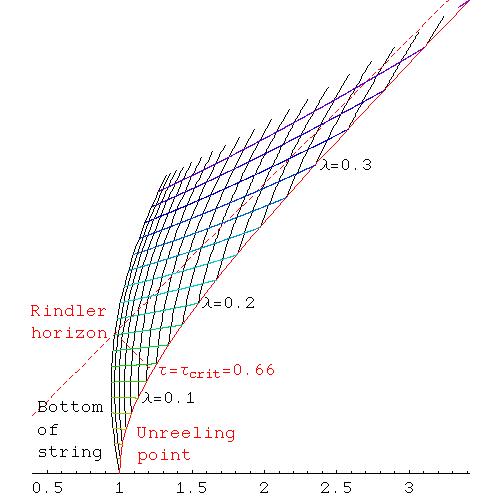The graph below shows the tension as a function of λ along a succession of spacelike slices.The results are quite close to the Newtonian values, for which the tension would simply be directly proportional to λ everywhere. For comparison, the tension in a static string (which matches the rate of unreeling to first order) is shown; this does not increase as rapidly with λ. Note that the limit on the tension set by the weak energy condition, (Q/K – 1), is 9.05.

Where the results depart most clearly from both the Newtonian case and the relativistic static string is in the acceleration of elements of the string. This is plotted below, for the same spacelike slices as the tension.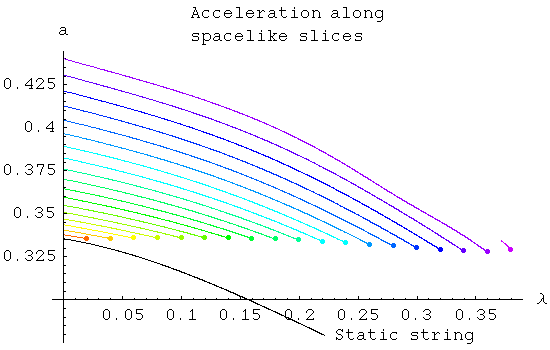Whereas the acceleration in the Newtonian case is both constant in time and identical for all elements of the string, and for a static string each element has a constant acceleration which is smaller for higher values of λ, in the case of constant unreeling, the acceleration of each element increases over time as well as decreasing along each spacelike slice.Although a string lowered in this fashion appears doomed to break eventually, this example shows that there’s no reason it can’t survive intact not only after τcrit, but also after the bottom of the string has passed through the horizon according to the string’s own definition of simultaneity. In other words, a spacelike slice orthogonal to the world lines of the string’s elements can run all the way from the bottom of the string, on the far side of the Rindler horizon, to the point of unreeling, with the tension everywhere remaining well below the limit set by the weak energy condition.

The animation on the left shows a string being unreeled at a constant rate, with R=0.2 and K=0.1. The distance shown vertically here is measured along the spacelike slices, so it is “proper distance” in the sense that these slices are everywhere orthogonal to the world lines of the elements of the string. The bands along the string are segments of 0.02 unstretched length; the animation shows the unreeling of a total unstretched length of 0.36. The red line that appears during the descent marks the Rindler horizon; the distance from the point of unreeling to the horizon, measured along these spacelike slices, is not fixed.

## Conclusions

What happens when an object is lowered across a horizon on the end of an elastic string? We have only been able to obtain exact solutions for two special cases: a perfectly slack string in free fall, and a string which is lowered in such a way as to be static in its own “flotilla” of accelerating frames. However, it seems likely that whenever an object is lowered across a horizon this way, the main qualitative features of those solutions will always be present:

• Given some function of proper time which expresses the rate at which the string is being unreeled, there will be a time τcrit after which no signal from the point of unreeling can reach the object at the end of the string before it passes through the horizon.
• After τcrit, the only way to keep the string from eventually breaking (assuming it hasn’t broken already) will be to continue to feed it out at an increasing rate. Clamping the string, attempting to pull the object back up, or even just feeding out the string at a constant rate, will all ultimately cause the string to break, though the exact time when that happens will depend on the physical properties of the string.

The Rindler metric for flat spacetime and its similarity to the Schwarzschild metric for black holes is discussed in Section 6.4 of General Relativity by Robert M. Wald.

### Notes

 A partial differential equation was constructed by describing the world lines of the elements of the string with functions T(τ, λ) and X(τ, λ); here λ identifies the particular element, τ varies along each world line (but is not necessarily proper time for the element), and T and X are the spacetime coordinates in some inertial frame. Given the assumption of a hyperelastic string — that is, a string obeying Hooke’s Law exactly — the stress-energy tensor was expressed in terms of the functions T and X, and the requirement that it be divergence-free produced a single partial differential equation in T and X: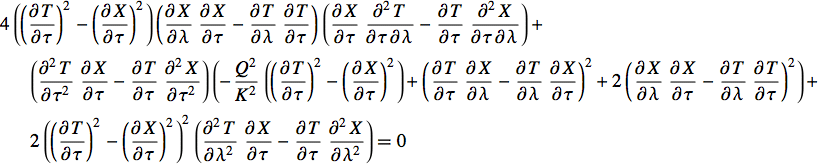To eliminate T, it was necessary to make a “choice of gauge” for τ. A convenient choice in this case was to define it to be the lapse in coordinate time since the unreeling of each element, making T simply:

T(τ, λ) = s2 sinh(λ / (R s2)) + τ

An approximate solution was found by setting X equal to a 32-order polynomial P in λ and τ, plus an initial offset equal to the x-coordinate of the point of unreeling:

X(τ, λ) = s2 cosh(λ / (R s2)) + P(τ, λ)

This made the boundary condition describing the way the string was unreeled very simple:

P(0, λ) = 0

The coefficients of the polynomial P were then found by requiring that all derivatives of the PDE up to order 32 were zero (at the point λ=0, τ=0), and that the tension at the bottom of the string was zero to the same order in τ. The solution was validated by comparing the purely geometrical proper acceleration of each world line with the acceleration required by the PDE; for the data displayed, these two values differ by less than 1%.

More details on the derivation of the PDE can be found in this supplementary page on relativistic elasticity.Science Notes / The Rindler Horizon / created Wednesday, 20 September 2006 / revised Monday, 16 July 2007